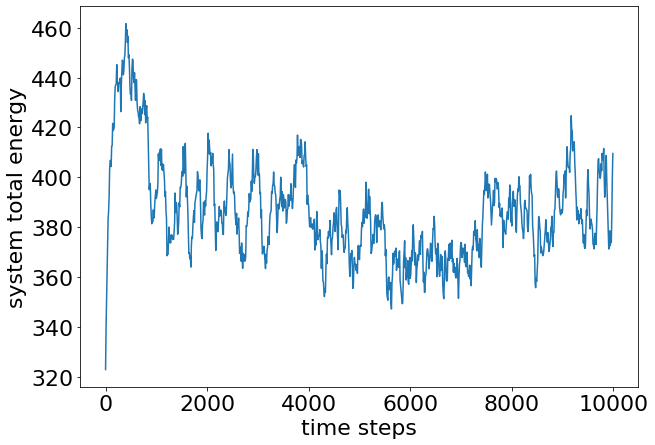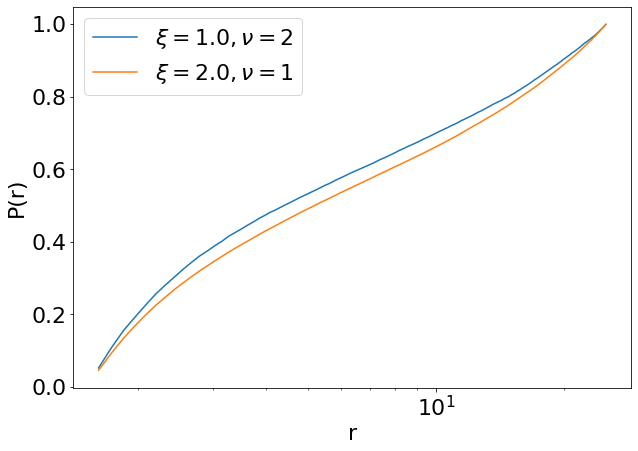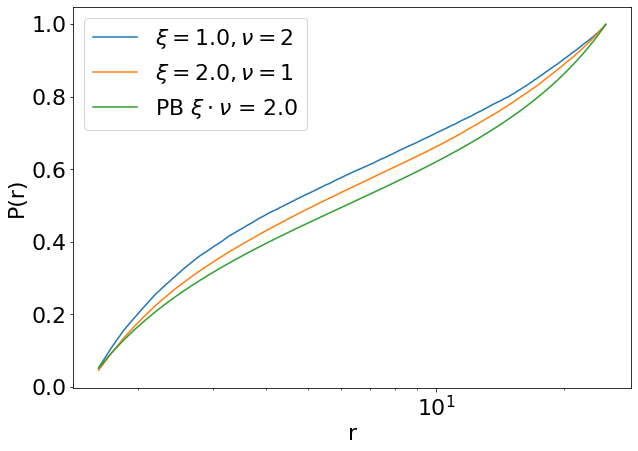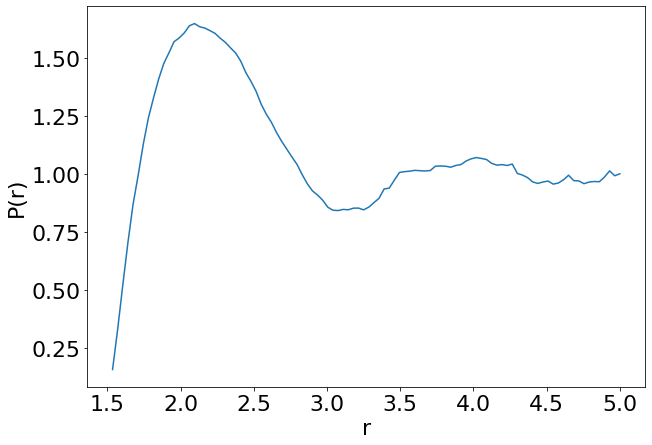# A Charged System: Counterion Condensation¶

## Introduction¶

In this tutorial, we simulate a charged system consisting of a fixed charged rod with ions around it. This setup represents a simplified model for polyelectrolyte gels. We will investigate the condensation of ions onto the oppositely charged rod and compare the results to a meanfield analytical solution obtained from Poisson−Boltzmann (PB) theory. Finally we will go beyond the expected applicability of PB and add concentrated additional salt ions to observe an overcharging effect.

The tutorial follows "Deserno, Markus, Christian Holm, and Sylvio May. "Fraction of condensed counterions around a charged rod: Comparison of Poisson−Boltzmann theory and computer simulations. Macromolecules 33.1 (2000): 199-206, 10.1021/ma990897o". We refer to that publication for further reading.

In :
import espressomd
espressomd.assert_features(['WCA', 'ELECTROSTATICS'])

from espressomd import System, interactions, electrostatics, observables, accumulators, math

import numpy as np
from scipy import optimize
%matplotlib inline
import matplotlib.pyplot as plt

In :
np.random.seed(41)
plt.rcParams.update({'font.size': 22})


# System setup¶

After importing the necessary ESPResSo features and external modules, we define a cubic system geometry and some physical parameters (which define our unit system).

In :
# system parameters
ROD_LENGTH = 50
BJERRUM_LENGTH = 1.0

# we assume a unit system where the elementary charge and the thermal energy are both 1
system = System(box_l=3 * [ROD_LENGTH])
KT = 1.
Q_E = 1.

system.time_step = 0.01
system.cell_system.skin = 0.4


We will build the charged rod from individual particles that are fixed in space. With this, we can use the particle-based electrostatics methods of ESPResSo. For analysis, we give the rod particles a different type than the counterions.

In :
# interaction parameters
WCA_EPSILON = 1.0
ION_DIAMETER = 1.0
# particle types
ROD_TYPE = 1
COUNTERION_TYPE = 2


Exercise:

• Setup the purely repulsive Weeks-Chandler-Anderson (WCA) interaction (Non-bonded Interactions) between the ions and between the ions and the rod particles. Use the parameters introduced in the cell above.

Hints:

• The WCA potential uses the same parameters as the Lennard-Jones potential, but the cutoff and shift are calculated automatically
• Use the Lorentz combining rule (arithmetic mean) to determine the sigma parameter of the interaction between the rod particles and the ions
In :
# ion-ion interaction
system.non_bonded_inter[COUNTERION_TYPE, COUNTERION_TYPE].wca.set_params(
epsilon=WCA_EPSILON, sigma=ION_DIAMETER)

# ion-rod interaction
system.non_bonded_inter[COUNTERION_TYPE, ROD_TYPE].wca.set_params(
epsilon=WCA_EPSILON, sigma=ION_DIAMETER / 2. + ROD_RADIUS)


Now we need to place the particles in the box

Exercise:

• Implement a function to place the rod particles along the $x_3$ axis in the middle of the simulation box and the ions randomly distributed
• Use the signature setup_rod_and_counterions(system, ion_valency, counterion_type, rod_charge_dens, N_rod_beads, rod_type)
• Determine the number of counterions from the condition of neutrality for the whole system (the rod should be positive, the counterions negative)
• Assign the rod particles and counterions their correct type
• Give the counterions a charge q according to their ion_valency
• Give the rod particles a charge such that the rod_charge_dens is uniformly distributed along the N_rod_beads individual particles
• Fix the rod particles in space so they do not get moved if forces act upon them
• Return the newly created counterion particles

Hints:

• Look into espresso particle properties to find the keywords to set charges and to fix particles
• use np.random.random() to generate the counterion positions
In :
def setup_rod_and_counterions(system, ion_valency, counterion_type,

# calculate charge of the single rod beads
rod_length = system.box_l
total_rod_charge = rod_charge_dens * rod_length

# number of counterions
N_ions = int(total_rod_charge / ion_valency)

rod_zs = np.linspace(0, rod_length, num=N_rod_beads, endpoint=False)
rod_positions = np.column_stack(([system.box_l / 2.] * N_rod_beads,
rod_zs))

ion_positions = np.random.random((N_ions, 3)) * system.box_l

counterion_type] * N_ions, q=[-ion_valency] * N_ions)

return counter_ions

In :
COUNTERION_VALENCY = 1
ROD_CHARGE_DENS = 2

# number of beads that make up the rod

setup_rod_and_counterions(system, COUNTERION_VALENCY, COUNTERION_TYPE,

# check that the particle setup was done correctly
assert abs(sum(system.part[:].q)) < 1e-10
assert np.all(system.part.select(type=ROD_TYPE).fix)


Now we set up the electrostatics method to calculate the forces and energies from the longrange coulomb interaction. ESPResSo uses so-called actors for electrostatics, magnetostatics and hydrodynamics. This ensures that unphysical combinations of algorithms are avoided, for example simultaneous usage of two electrostatic interactions. Adding an actor to the system also activates the method and calls necessary initialization routines. Here, we define a P$^3$M object using the Bjerrum length and rms force error. This automatically starts a tuning function which tries to find optimal parameters for P$^3$M and prints them to the screen. For more details, see the Espresso documentation.

In :
p3m_params = {'prefactor': KT * BJERRUM_LENGTH * Q_E**2,
'accuracy': 1e-3}


For the accuracy, ESPResSo estimates the relative error in the force calculation introduced by the approximations of $P^3M$. We choose a relatively poor accuracy (large value) for this tutorial to make it run faster. For your own production simulations you should reduce that number.

Exercise:

• Set up a p3m instance and add it to the actors of the system
In :
p3m = electrostatics.P3M(**p3m_params)


Before we can start the simulation, we need to remove the overlap between particles to avoid large forces which would crash the simulation. For this, we use the steepest descent integrator with a relative convergence criterion for forces and energies.

In :
def remove_overlap(system, sd_params):
# Removes overlap by steepest descent until forces or energies converge
# Set up steepest descent integration
system.integrator.set_steepest_descent(f_max=0,
gamma=sd_params['damping'],
max_displacement=sd_params['max_displacement'])

# Initialize integrator to obtain initial forces
system.integrator.run(0)
maxforce = np.max(np.linalg.norm(system.part[:].f, axis=1))
energy = system.analysis.energy()['total']

i = 0
while i < sd_params['max_steps'] // sd_params['emstep']:
prev_maxforce = maxforce
prev_energy = energy
system.integrator.run(sd_params['emstep'])
maxforce = np.max(np.linalg.norm(system.part[:].f, axis=1))
relforce = np.abs((maxforce - prev_maxforce) / prev_maxforce)
energy = system.analysis.energy()['total']
relener = np.abs((energy - prev_energy) / prev_energy)
if i > 1 and (i + 1) % 4 == 0:
print(f"minimization step: {(i+1)*sd_params['emstep']:4.0f}"
f"    max. rel. force change:{relforce:+3.3e}"
f"    rel. energy change:{relener:+3.3e}")
if relforce < sd_params['f_tol'] or relener < sd_params['e_tol']:
break
i += 1

system.integrator.set_vv()

In :
STEEPEST_DESCENT_PARAMS = {'f_tol': 1e-2,
'e_tol': 1e-5,
'damping': 30,
'max_steps': 10000,
'max_displacement': 0.01,
'emstep': 10}

remove_overlap(system, STEEPEST_DESCENT_PARAMS)

minimization step:   40    max. rel. force change:+4.117e-02    rel. energy change:+5.690e-03
minimization step:   80    max. rel. force change:+4.860e-02    rel. energy change:+5.895e-03
minimization step:  120    max. rel. force change:+1.574e-01    rel. energy change:+6.268e-03
minimization step:  160    max. rel. force change:+1.294e-01    rel. energy change:+6.106e-03
minimization step:  200    max. rel. force change:+3.497e-02    rel. energy change:+5.924e-03
minimization step:  240    max. rel. force change:+4.085e-02    rel. energy change:+6.396e-03
minimization step:  280    max. rel. force change:+4.912e-02    rel. energy change:+6.965e-03
minimization step:  320    max. rel. force change:+6.108e-02    rel. energy change:+7.678e-03
minimization step:  360    max. rel. force change:+6.878e-02    rel. energy change:+7.350e-03
minimization step:  400    max. rel. force change:+6.456e-02    rel. energy change:+7.315e-03
minimization step:  440    max. rel. force change:+8.429e-02    rel. energy change:+8.290e-03
minimization step:  480    max. rel. force change:+4.140e-02    rel. energy change:+7.379e-03
minimization step:  520    max. rel. force change:+4.895e-02    rel. energy change:+8.189e-03
minimization step:  560    max. rel. force change:+6.028e-02    rel. energy change:+9.254e-03
minimization step:  600    max. rel. force change:+5.567e-02    rel. energy change:+9.819e-03


After the overlap is removed, we activate a thermostat to simulate the system at a given temperature.

In :
LANGEVIN_PARAMS = {'kT': KT,
'gamma': 0.5,
'seed': 42}
system.thermostat.set_langevin(**LANGEVIN_PARAMS)


## First run and observable setup¶

Before running the simulations to obtain the histograms, we need to decide how long we need to equilibrate the system. For this we plot the total energy vs the time steps.

In :
energies = []
STEPS_PER_SAMPLE_FIRST_RUN = 10
N_SAMPLES_FIRST_RUN = 1000
for i in range(N_SAMPLES_FIRST_RUN):
system.integrator.run(STEPS_PER_SAMPLE_FIRST_RUN)
energies.append(system.analysis.energy()['total'])

In :
# plot time in time_steps so we can judge the number of warmup steps
ts = np.arange(0, N_SAMPLES_FIRST_RUN) * STEPS_PER_SAMPLE_FIRST_RUN
plt.figure(figsize=(10, 7))
plt.plot(ts, energies)
plt.xlabel('time steps')
plt.ylabel('system total energy')
plt.show()In :
WARMUP_STEPS = 5000
STEPS_PER_SAMPLE = 100


Now we are ready to implement the observable calculation. As we are interested in the condensation of counterions on the rod, the physical quantity of interest is the density of charges $\rho(r)$ around the rod, where $r$ is the distance from the rod. We need many samples to calculate the density from histograms.

From the last tutorial you should already be familiar with the concepts of observables and accumulators in ESPResSo. We will use the CylindricalDensityProfile observable and the MeanVarianceCalculator accumulator

Exercise:

• Write a function setup_profile_calculation(system, delta_N, ion_types, r_min, n_radial_bins) to create observables for $\rho(r)$
• delta_N is the number of integration steps between observable calculation
• ion_types is a list of types for which the radial distances should be calculated. For the moment we only have counterions, but later we will also add additional salt ions for which we would also like to calculate the density
• return a a dictionary of the accumulators radial_distances[counterion_type] = <accumulator> and the edges of the bins

Hints:

• Use system.part.select(type=...) to get only the particles of a specific type
• The azimuthal angle and the $x_3$ position are irrelevant, so you need only one big bin for these coordinates
In :
def setup_profile_calculation(system, delta_N, ion_types, r_min, n_radial_bins):
ctp = math.CylindricalTransformationParameters(center = np.array(system.box_l) / 2.,
axis = [0, 0, 1],
orientation = [1, 0, 0])
for ion_type in ion_types:
ion_ids = system.part.select(type=ion_type).id
ids=ion_ids,
transform_params = ctp,
min_r=r_min,
min_z=-system.box_l / 2.,
max_r=system.box_l / 2.,
max_z=system.box_l / 2.)


In :
r_min = ROD_RADIUS + ION_DIAMETER / 2.
r_max = system.box_l / 2.
assert isinstance(
assert len(bin_edges) == N_RADIAL_BINS + 1


To run the simulation with different parameters, we need a way to reset the system and return it to an empty state before setting it up again.

Exercise:

• Write a function clear_system(system) that
• turns off the thermostat
• removes all particles
• removes all accumulators added to the auto-update-list
• resets the system clock

Hints:

In :
def clear_system(system):
system.thermostat.turn_off()
system.part.clear()
system.auto_update_accumulators.clear()
system.time = 0.

In :
clear_system(system)


## Production run and analysis¶

Now we are finally ready to run the simulations and produce the data we can compare to the Poisson-Boltzmann predictions. First we define the parameters and then loop over them.

In :
runs = [{'params': {'counterion_valency': 2, 'rod_charge_dens': 1},
'histogram': None},
{'params': {'counterion_valency': 1, 'rod_charge_dens': 2},
'histogram': None}
]
N_SAMPLES = 1500


For longer simulation runs it will be convenient to have a progress bar

In :
def integrate_system(system, n_steps):
for i in range(100):
system.integrator.run(int(n_steps / 100))
print(f'progress={i}%', end='\r')
print('progress=100%')


Exercise:

• Run the simulation for the parameters given above and save the histograms in the corresponding dictionary for analysis

Hints:

• Don't forget to clear the system before setting up the system with a new set of parameters
• Don't forget to tune() the p3m instance after each change of parameters. If we reuse the p3m that was tuned before, likely the desired accuracy will not be achieved.
• Extract the radial density profile from the accumulator via .mean()
In :
for run in runs:
clear_system(system)
setup_rod_and_counterions(
system, run['params']['counterion_valency'], COUNTERION_TYPE,
p3m.tune()
remove_overlap(system, STEEPEST_DESCENT_PARAMS)
system.thermostat.set_langevin(**LANGEVIN_PARAMS)
print('starting warmup')
integrate_system(system, WARMUP_STEPS)
print('starting simulation')
integrate_system(system, N_SAMPLES * STEPS_PER_SAMPLE)

print('simulation for parameters {} done\n'.format(run['params']))

minimization step:   40    max. rel. force change:+2.955e-02    rel. energy change:+6.903e-03
minimization step:   80    max. rel. force change:+3.465e-02    rel. energy change:+6.025e-03
minimization step:  120    max. rel. force change:+4.101e-02    rel. energy change:+5.899e-03
minimization step:  160    max. rel. force change:+4.912e-02    rel. energy change:+6.142e-03
minimization step:  200    max. rel. force change:+6.052e-02    rel. energy change:+6.782e-03
starting warmup
progress=100%
starting simulation
progress=100%
simulation for parameters {'counterion_valency': 2, 'rod_charge_dens': 1} done

minimization step:   40    max. rel. force change:+7.683e-01    rel. energy change:+4.829e-01
minimization step:   80    max. rel. force change:+9.299e-01    rel. energy change:+7.919e-03
minimization step:  120    max. rel. force change:+5.162e-02    rel. energy change:+5.518e-03
minimization step:  160    max. rel. force change:+7.904e-02    rel. energy change:+5.603e-03
minimization step:  200    max. rel. force change:+5.228e-01    rel. energy change:+5.219e-03
minimization step:  240    max. rel. force change:+5.482e-02    rel. energy change:+5.424e-03
minimization step:  280    max. rel. force change:+1.176e-01    rel. energy change:+5.357e-03
minimization step:  320    max. rel. force change:+3.329e-02    rel. energy change:+5.599e-03
minimization step:  360    max. rel. force change:+3.781e-02    rel. energy change:+6.033e-03
minimization step:  400    max. rel. force change:+4.445e-02    rel. energy change:+6.597e-03
minimization step:  440    max. rel. force change:+5.372e-02    rel. energy change:+7.312e-03
minimization step:  480    max. rel. force change:+1.645e-02    rel. energy change:+7.973e-03
minimization step:  520    max. rel. force change:+8.291e-02    rel. energy change:+7.884e-03
minimization step:  560    max. rel. force change:+1.518e-01    rel. energy change:+7.821e-03
minimization step:  600    max. rel. force change:+5.497e-02    rel. energy change:+8.166e-03
minimization step:  640    max. rel. force change:+4.654e-02    rel. energy change:+8.431e-03
minimization step:  680    max. rel. force change:+5.573e-02    rel. energy change:+9.529e-03
minimization step:  720    max. rel. force change:+7.556e-02    rel. energy change:+9.424e-03
minimization step:  760    max. rel. force change:+2.378e-01    rel. energy change:+9.353e-03
minimization step:  800    max. rel. force change:+5.963e-02    rel. energy change:+9.904e-03
minimization step:  840    max. rel. force change:+5.344e-02    rel. energy change:+1.099e-02
minimization step:  880    max. rel. force change:+3.410e-02    rel. energy change:+1.277e-02
minimization step:  920    max. rel. force change:+1.746e-02    rel. energy change:+1.128e-02
minimization step:  960    max. rel. force change:+6.482e-02    rel. energy change:+1.061e-02
minimization step: 1000    max. rel. force change:+6.106e-02    rel. energy change:+1.127e-02
minimization step: 1040    max. rel. force change:+4.753e-02    rel. energy change:+1.079e-02
minimization step: 1080    max. rel. force change:+5.622e-02    rel. energy change:+1.257e-02
minimization step: 1120    max. rel. force change:+9.735e-02    rel. energy change:+1.463e-02
minimization step: 1160    max. rel. force change:+3.547e-02    rel. energy change:+1.207e-02
minimization step: 1200    max. rel. force change:+5.549e-02    rel. energy change:+1.233e-02
minimization step: 1240    max. rel. force change:+6.687e-02    rel. energy change:+1.460e-02
minimization step: 1280    max. rel. force change:+7.411e-02    rel. energy change:+1.666e-02
minimization step: 1320    max. rel. force change:+6.887e-02    rel. energy change:+1.777e-02
minimization step: 1360    max. rel. force change:+6.912e-02    rel. energy change:+1.880e-02
minimization step: 1400    max. rel. force change:+3.036e-02    rel. energy change:+1.927e-02
minimization step: 1440    max. rel. force change:+7.139e-02    rel. energy change:+1.858e-02
minimization step: 1480    max. rel. force change:+4.626e-02    rel. energy change:+1.927e-02
minimization step: 1520    max. rel. force change:+7.423e-02    rel. energy change:+2.195e-02
minimization step: 1560    max. rel. force change:+3.333e-02    rel. energy change:+2.259e-02
minimization step: 1600    max. rel. force change:+4.441e-03    rel. energy change:+2.359e-02
starting warmup
progress=100%
starting simulation
progress=100%
simulation for parameters {'counterion_valency': 1, 'rod_charge_dens': 2} done



Question

• Why does the second simulation take much longer than the first one?

The rod charge density is doubled, so the total charge of the counterions needs to be doubled, too. Since their valency is only half of the one in the first run, there will be four times more counterions in the second run.

We plot the density of counterions around the rod as the normalized integrated radial counterion charge distribution function $P(r)$, meaning the integrated probability to find an amount of charge within the radius $r$. We express the rod charge density $\lambda$ in terms of the dimensionless Manning parameter $\xi = \lambda l_B / e$ where $l_B$ is the Bjerrum length and $e$ the elementary charge

In :
# With the notion of P(r) the probability to find the charge up to r,
# we only use the right side of the bin edges for plotting
rs = bin_edges[1:, 0, 0, 0]

fig, ax = plt.subplots(figsize=(10, 7))
for run in runs:
hist = np.array(run['histogram'][:, 0, 0])
# The CylindricalDensityProfile normalizes the bin values by the bin size.
# We want the 'raw' distribution (number of ions within a radius)
# so we need to multiply by the radii
hist = hist * rs
cum_hist = np.cumsum(hist)
cum_hist /= cum_hist[-1]
manning_xi = run['params']['rod_charge_dens'] * BJERRUM_LENGTH / Q_E
ax.plot(rs, cum_hist, label=r'$\xi ={}, \nu = {}$'.format(manning_xi,
run['params']['counterion_valency']))
ax.set_xscale('log')
ax.legend()
plt.xlabel('r')
plt.ylabel('P(r)')
plt.show()In the semilogarithmic plot we see an inflection point of the cumulative charge distribution which is the indicator for ion condensation. To compare to the meanfield approach of PB, we calculate the solution of the analytical expressions given in 10.1021/ma990897o

In :
def eq_to_solve_for_gamma(gamma, manning_parameter, rod_radius, max_radius):
# eq 7 - eq 6 from 10.1021/ma990897o
return gamma * np.log(max_radius / rod_radius) - np.arctan(1 / gamma) + np.arctan((1 - manning_parameter) / gamma)

# eq 7 from 10.1021/ma990897o
return max_radius * np.exp(-np.arctan(1. / gamma) / gamma)

# eq 8 and 9 from 10.1021/ma990897o
return 1. / manning_parameter + gamma / manning_parameter * np.tan(gamma * np.log(r / manning_radius))


For multivalent counterions, the manning parameter $\xi$ has to be multiplied by the valency $\nu$. The result depends only on the product of rod_charge_dens and ion_valency, so we only need one curve

In :
rod_charge_density = runs['params']['rod_charge_dens']
ion_valency = runs['params']['counterion_valency']
manning_parameter_times_valency = BJERRUM_LENGTH * rod_charge_density * ion_valency

gamma = optimize.fsolve(eq_to_solve_for_gamma, 1, args=(
manning_parameter_times_valency, r_min, r_max))

PB_probability = calc_PB_probability(

ax.plot(rs, PB_probability, label=r'PB $\xi \cdot \nu$ = {}'.format(
manning_parameter_times_valency))
ax.legend()
ax.set_xscale('log')
fig

Out:We see that overall agreement is quite good, but the deviations from the PB solution get stronger the more charged the ions are. Poisson Boltzmann makes two simplifying assumptions: Particles are points and there are no correlations between the particles. Both is not given in the simulation. Excluded volume effects can only lower the density, but we see in the figure that the simulated density is always larger that the calculated one. This means that correlation effects cause the discrepancy.

Above simulations were performed for a system where all ions come from dissociation off the polyelectrolyte. We can also investigate systems where there are additional salt ions present.

In :
def add_salt(system, anion_params, cation_params):

N_anions = anion_params['number']
N_cations = cation_params['number']

anion_positions = np.random.random((N_anions, 3)) * system.box_l
cation_positions = np.random.random((N_cations, 3)) * system.box_l

anions = system.part.add(pos=anion_positions, type=[anion_params['type']] * N_anions,
q=[-anion_params['valency']] * N_anions)
cations = system.part.add(pos=cation_positions, type=[cation_params['type']] * N_cations,
q=[cation_params['valency']] * N_cations)

return anions, cations

In :
ANION_PARAMS = {'type': 3,
'valency': 2,
'number': 150}
CATION_PARAMS = {'type': 4,
'valency': 2,
'number': 150}
ROD_LENGTH = 10
ROD_CHARGE_DENS = 1
COUNTERION_VALENCY = 1

STEPS_PER_SAMPLE_SALT = 20
N_SAMPLES_SALT = 1500

all_ion_types = [COUNTERION_TYPE, ANION_PARAMS['type'], CATION_PARAMS['type']]

In :
# set interactions of salt with the rod and all ions
for salt_type in [ANION_PARAMS['type'], CATION_PARAMS['type']]:
system.non_bonded_inter[salt_type, ROD_TYPE].wca.set_params(
epsilon=WCA_EPSILON, sigma=ION_DIAMETER / 2. + ROD_RADIUS)
for ion_type in all_ion_types:
system.non_bonded_inter[salt_type, ion_type].wca.set_params(
epsilon=WCA_EPSILON, sigma=ION_DIAMETER)

In :
clear_system(system)
system.box_l = 3 * [ROD_LENGTH]
counterions = setup_rod_and_counterions(
system, COUNTERION_VALENCY, COUNTERION_TYPE,
anions, cations = add_salt(system, ANION_PARAMS, CATION_PARAMS)
assert abs(sum(anions.q) + sum(cations.q)) < 1e-10

p3m.tune()
remove_overlap(system, STEEPEST_DESCENT_PARAMS)
system.thermostat.set_langevin(**LANGEVIN_PARAMS)
print('starting warmup')
integrate_system(system, WARMUP_STEPS)
print('starting simulation')
integrate_system(system, N_SAMPLES_SALT * STEPS_PER_SAMPLE_SALT)
print('simulation done')

minimization step:   40    max. rel. force change:+9.562e-01    rel. energy change:+9.299e-01
minimization step:   80    max. rel. force change:+7.655e-01    rel. energy change:+1.977e-01
minimization step:  120    max. rel. force change:+2.123e-01    rel. energy change:+4.752e-03
minimization step:  160    max. rel. force change:+8.306e-03    rel. energy change:+2.150e-03
starting warmup
progress=100%
starting simulation
progress=100%
simulation done

In :
rs = bin_edges[1:, 0, 0, 0]
cum_hists = {}
for ion_type in all_ion_types:
hist = hist[:, 0, 0] * rs
cum_hist = np.cumsum(hist)
cum_hist /= cum_hist[-1]
cum_hists[ion_type] = cum_hist


Exercise:

• Use the cumulative histograms from the cell above to create the cumulative charge histogram of the total ion charge

Hints

• You need to account for the fact that the cumulative histograms are all normalized, but the total charge of each ion type is different
In :
counterion_charge = sum(counterions.q)
anion_charge = sum(anions.q)
cation_charge = sum(cations.q)
charge_hist = counterion_charge * cum_hists[COUNTERION_TYPE] + \
anion_charge * cum_hists[ANION_PARAMS['type']] + \
cation_charge * cum_hists[CATION_PARAMS['type']]

In :
charge_hist /= charge_hist[-1]
fig2, ax2 = plt.subplots(figsize=(10, 7))
ax2.plot(rs, charge_hist)
ax2.set_xscale('linear')
plt.xlabel('r')
plt.ylabel('P(r)')
plt.show()You should observe a strong overcharging effect, where ions accumulate close to the rod.